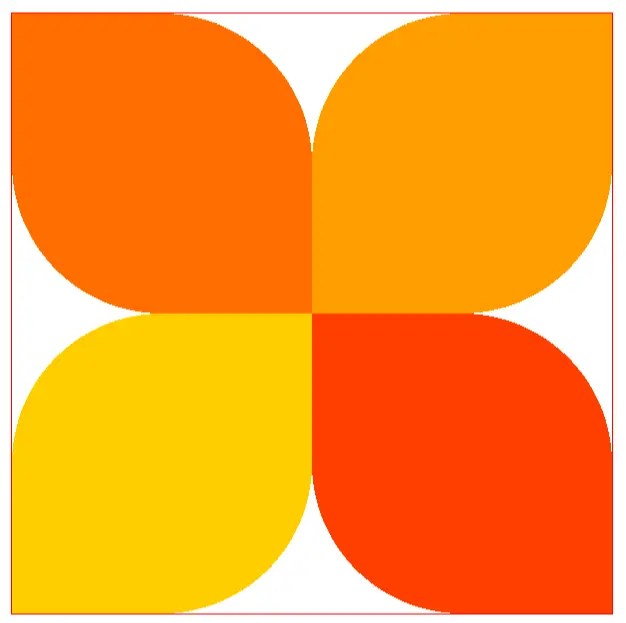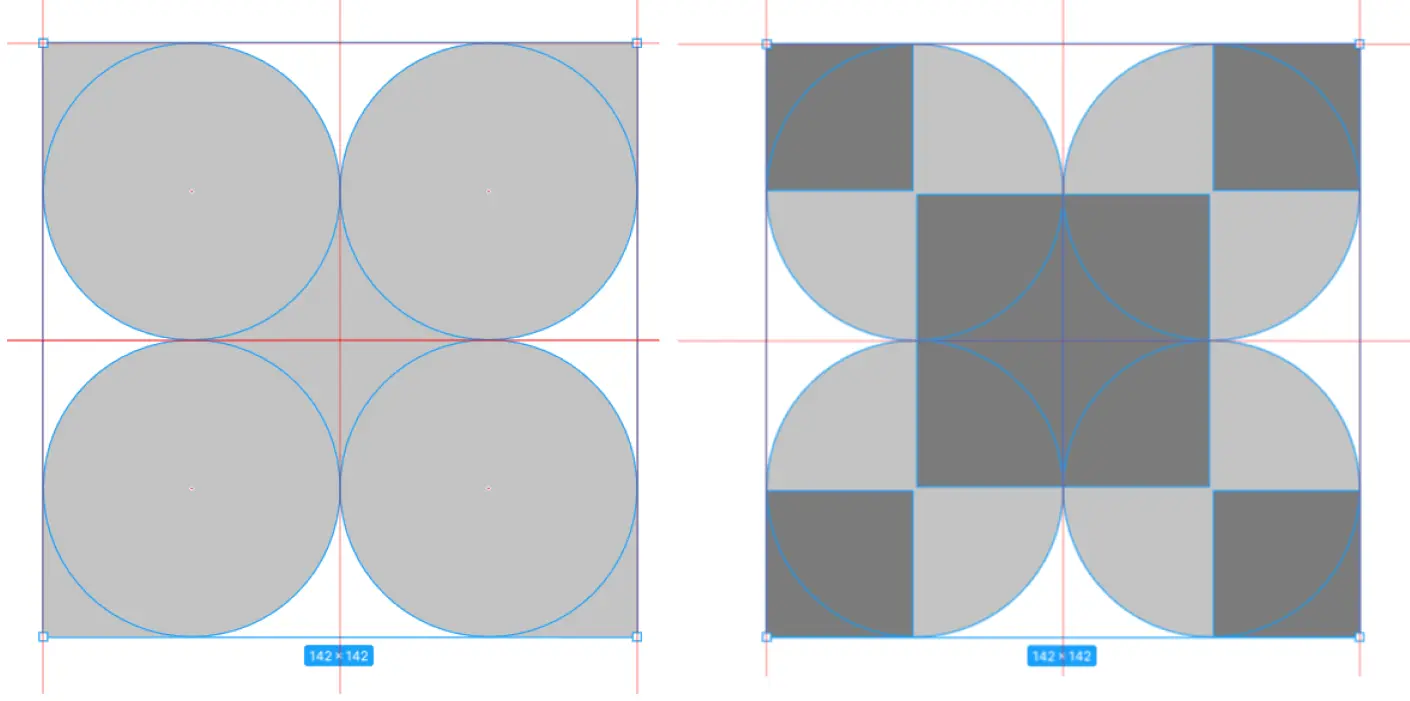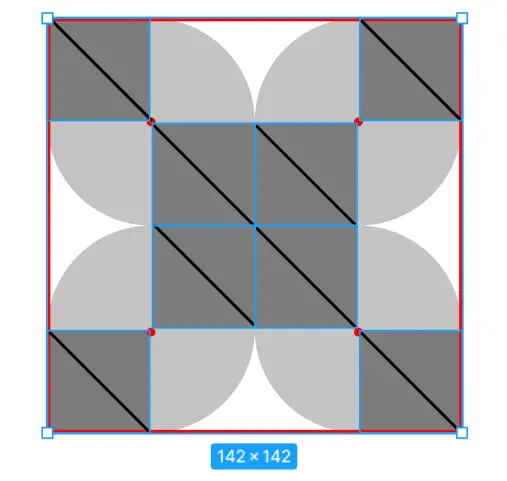# To draw a square in CSS linear gradient, think of triangles

Ayyash
·Mar 9, 2022·

The easiest way to draw a pattern in CSS for linear-gradient that produces squares, is to think of triangles. A linear gradient that starts in a corner and stops at a certain percentage is doable. But a linear vertical or horizontal gradient cannot be controlled in both directions, only one. So visually, if I want to create this pattern:Modeling looks like this, the circle radials are easy to produce, but the squares are tougher.So triangles (I choose this because it's the simplest but the options are unlimited)And the result is in CodePen